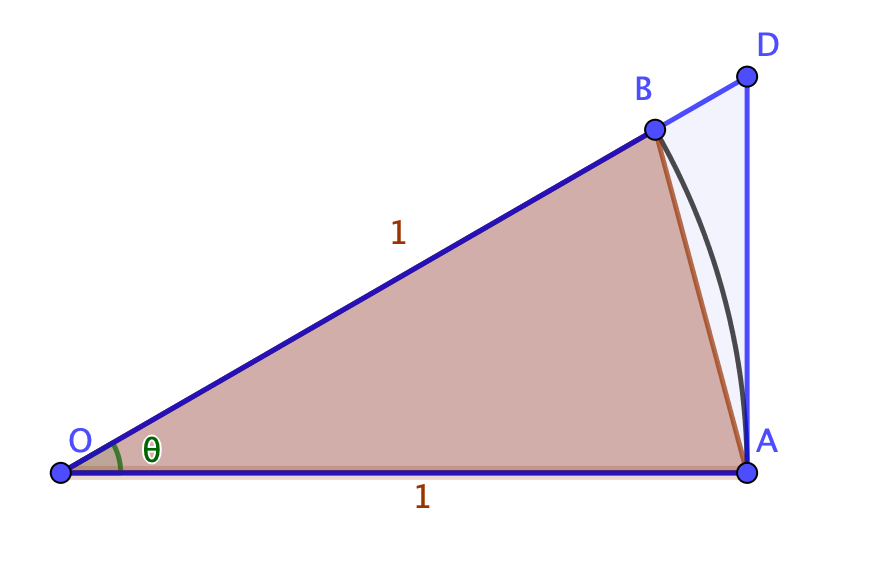Every so often, I get a misguided message from someone claiming that the value we use for $\pi$ is wrong. (I try not to dunk on such messages, tempting as it is. But really, if you think everyone who’s looked at it, from Archimedes to Newton, Euler, Ramanujan and Gosper, has got the same wrong answer by different methods while you alone have it right, it might be worth adjusting your humility settings a bit.)

In any event, here’s one way of finding the value of $\pi$ using largely A-level methods.

### Comparing shapesIt doesn’t really matter what the value of $\theta$ is, we’re trying to compare the areas of some shapes. There are three we care about:

• The smallest is the red triangle OAB, which has area $\frac{1}{2}\br{1}\br{\sin(\theta)}$;
• The middle area is the circular segment OAB, which has area $\frac{1}{2}\br{1^2}\theta$;
• The largest is the blue triangle OAD, which has area $\frac{1}{2}\br{1}\br{\tan(\theta)}$ (treating OA as the base and noting that AOD is a right angle).

That gives us an inequality: $\frac{1}{2}\sin(\theta) < \frac{1}{2}\theta < \frac{1}{2}\tan(\theta)$, at least when $0 < \theta < \piby 2$.

Double all of that to get it in its neatest form: $\sin(\theta) < \theta < \tan(\theta)$.

This is true for any $\theta$ in the first quadrant – so we can pick any angle where we know $\sin(\theta)$ and $\tan(\theta)$ to start from.

For example: if you pick $\theta = \piby 4$, you get $\frac{1}{\sqrt{2}} < \piby 4 < 1$, or $2\sqrt{2} < \pi < 4$. An earthshattering result, I think you’ll agree: we’ve managed to pin down $\pi$ to “probably between 3 and 4”.

Of course, we’re just getting started.

### Splitting the angle in half

The thing is, if we could work out the value of $\tan\br{\frac{1}{2}\theta}$ and $\sin\br{\frac{1}{2}\theta}$, we could work out the area of triangles much closer to the circle and get better bounds on $\pi$.

And, as it turns out, we can! Of course we can.

Let $T = \tan(\theta)$ and $t = \tan\br{\frac{1}{2}\theta}$. Then, using the double-angle formula, $T = \frac{2t}{1-t^2}$ – and we know what $T$ is. (It’s currently 1, but we’re going to solve it in general.)

Rearranging gives $Tt^2 + 2t - T = 0$. You could, of course, throw the formula at this; I prefer:

• Multiply by $T$: $T^2t^2 + 2tT - T^2 = 0$
• Complete the square: $\br{Tt + 1}^2 = 1 + T^2$
• Finish it off: $t = \frac{\sqrt{1+T^2} - 1}{T}$

So we can readily get $t$ in terms of $T$. What about $s$?

(I should say: $s$ is $\sin\br{\frac{1}{2}\theta}$. $S$ is $\sin(\theta)$. And $c = \cos\br{\frac{1}{2}\theta}$, which will come in useful; we don’t need to work $c$ out.)

There’s a way to do it with just the value of $S$, but it’s considerably easier if you involve the $t$ you’ve just worked out: since $S = 2sc$, and $t = \frac{s}{c}$, $S = \frac{2s^2}{t}$.

A quick rearrange gives $s = \sqrt{\frac{St}{2}}$.

So, taking our original values, we can work out that splitting the angle in half gives $\tan\br{\piby8} = \sqrt{2}-1$ and $\sin\br{\piby8} = \sqrt{\frac{\sqrt{2}-1}{2\sqrt{2}}}$.

Using our inequality from before and multiplying by 8 gives (with a bit of work) $4\br{2-\sqrt{2}} < \pi < 8\sqrt{2} - 8$ – so now we have it between 3.06 and 3.32 or so, which is considerably better.

### A pair of sequences!

We could repeat this as often as we wanted to (or could be bothered).

More formally, if we define the sequences:

• $t_k = \frac{\sqrt{1+t_{k-1}}-1}{t_{k-1}}$ for $k \ge 3$ and with $t_2 = 1$; and
• $s_k = \sqrt{\frac{s_{k-1}t_k}{2}}$ for $k \ge 3$ and with $s_2 = \frac{1}{\sqrt{2}}$

… then the inequality $2^{k} s_k < \pi < 2^k t_k$ holds for all $k \ge 2$.

### … and they get closer together

As $k$ gets larger, $t_k$ gets smaller - with the result that $t_k \approx \frac{1}{2}t_{k-1}$.

Consider $R_k=\frac{s_k}{t_k}$, which (by definition of $s_k$) is $\sqrt{\frac{s_{k-1}}{2t_k}}$. But since $2t_k \approx t_{k-1}$, we find $R \approx \sqrt{\frac{s_{k-1}}{t_{k-1}}}$. This means that $R_k$ is closer to 1 than $R_{k-1}$ is, and approaches 1 in the limit as $k$ gets large.

So: this sequence will give progressively closer values for lower and upper bounds of $\pi$, and can be used to get as close a value as you have the patience for!

What’s your favourite way to calculate the value of $\pi$? Bonus points if you can explain geometrically what’s going on!